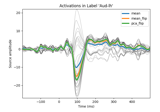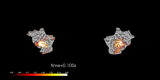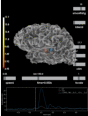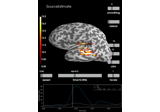# mne.VectorSourceEstimate¶

class mne.VectorSourceEstimate(data, vertices=None, tmin=None, tstep=None, subject=None, verbose=None)[source]

Container for vector surface source estimates.

For each vertex, the magnitude of the current is defined in the X, Y and Z directions.

Parameters
dataarray of shape (n_dipoles, 3, n_times)

The data in source space. Each dipole contains three vectors that denote the dipole strength in X, Y and Z directions over time.

verticeslist of array, shape (2,)

Vertex numbers corresponding to the data. The first element of the list contains vertices of left hemisphere and the second element contains vertices of right hemisphere.

tminfloat

Time point of the first sample in data.

tstepfloat

Time step between successive samples in data.

subject

The subject name. While not necessary, it is safer to set the subject parameter to avoid analysis errors.

verbose

If not None, override default verbose level (see mne.verbose() and Logging documentation for more). If used, it should be passed as a keyword-argument only.

SourceEstimate

A container for surface source estimates.

VolSourceEstimate

A container for volume source estimates.

MixedSourceEstimate

A container for mixed surface + volume source estimates.

Notes

New in version 0.15.

Attributes
subject

The subject name.

timesarray of shape (n_times,)

A timestamp for each sample.

shapetuple

Shape of the data.

Methods

 Add source estimates. Divide source estimates. __hash__(/) Return hash(self). Multiply source estimates. Negate the source estimate. Subtract source estimates. bin(width[, tstart, tstop, func]) Return a source estimate object with data summarized over time bins. Return copy of source estimate instance. crop([tmin, tmax, include_tmax]) Restrict SourceEstimate to a time interval. expand(vertices) Expand SourceEstimate to include more vertices. extract_label_time_course(labels, src[, …]) Extract label time courses for lists of labels. get_peak([hemi, tmin, tmax, mode, …]) Get location and latency of peak amplitude. in_label(label) Get a source estimate object restricted to a label. Compute magnitude of activity without directionality. Make a summary stc file with mean over time points. normal(**kwargs) Warning DEPRECATED: stc.normal(src) is deprecated and will be removed in 0.22, use stc.project(“normal”, src) instead plot([subject, hemi, colormap, time_label, …]) Plot VectorSourceEstimate with PySurfer. project(directions[, src, use_cps]) Project the data for each vertex in a given direction. resample(sfreq[, npad, window, n_jobs, verbose]) Resample data. save(fname[, ftype, verbose]) Save the full source estimate to an HDF5 file. Take the square root. Make a summary stc file with sum over time points. time_as_index(times[, use_rounding]) Convert time to indices. to_data_frame([index, scalings, …]) Export data in tabular structure as a pandas DataFrame. to_original_src(src_orig[, subject_orig, …]) Get a source estimate from morphed source to the original subject. transform(func[, idx, tmin, tmax, copy]) Apply linear transform. transform_data(func[, idx, tmin_idx, tmax_idx]) Get data after a linear (time) transform has been applied.
__add__(a)[source]

__div__(a)[source]

Divide source estimates.

__mul__(a)[source]

Multiply source estimates.

__neg__()[source]

Negate the source estimate.

__sub__(a)[source]

Subtract source estimates.

bin(width, tstart=None, tstop=None, func=<function mean>)[source]

Return a source estimate object with data summarized over time bins.

Time bins of width seconds. This method is intended for visualization only. No filter is applied to the data before binning, making the method inappropriate as a tool for downsampling data.

Parameters
widthscalar

Width of the individual bins in seconds.

tstart

Time point where the first bin starts. The default is the first time point of the stc.

tstop

Last possible time point contained in a bin (if the last bin would be shorter than width it is dropped). The default is the last time point of the stc.

funccallable()

Function that is applied to summarize the data. Needs to accept a numpy.array as first input and an axis keyword argument.

Returns
stc

The binned source estimate.

copy()[source]

Return copy of source estimate instance.

Returns
stcinstance of SourceEstimate

A copy of the source estimate.

crop(tmin=None, tmax=None, include_tmax=True)[source]

Restrict SourceEstimate to a time interval.

Parameters
tmin

The first time point in seconds. If None the first present is used.

tmax

The last time point in seconds. If None the last present is used.

include_tmaxbool

If True (default), include tmax. If False, exclude tmax (similar to how Python indexing typically works).

New in version 0.19.

Returns
stcinstance of SourceEstimate

The cropped source estimate.

property data

Numpy array of source estimate data.

expand(vertices)[source]

Expand SourceEstimate to include more vertices.

This will add rows to stc.data (zero-filled) and modify stc.vertices to include all vertices in stc.vertices and the input vertices.

Parameters
vertices

New vertices to add. Can also contain old values.

Returns
stc

The modified stc (note: method operates inplace).

extract_label_time_course(labels, src, mode='auto', allow_empty=False, verbose=None)[source]

Extract label time courses for lists of labels.

This function will extract one time course for each label. The way the time courses are extracted depends on the mode parameter.

Parameters
labels

If using a surface or mixed source space, this should be the Label’s for which to extract the time course. If working with whole-brain volume source estimates, this must be one of:

• a string path to a FreeSurfer atlas for the subject (e.g., their ‘aparc.a2009s+aseg.mgz’) to extract time courses for all volumes in the atlas

• a two-element list or tuple, the first element being a path to an atlas, and the second being a list or dict of volume_labels to extract (see mne.setup_volume_source_space() for details).

Changed in version 0.21.0: Support for volume source estimates.

srcinstance of SourceSpaces

The source spaces for the source time courses.

modestr

Extraction mode, see Notes.

allow_empty

False (default) will emit an error if there are labels that have no vertices in the source estimate. True and 'ignore' will return all-zero time courses for labels that do not have any vertices in the source estimate, and True will emit a warning while and “ignore” will just log a message.

Changed in version 0.21.0: Support for “ignore”.

verbose

If not None, override default verbose level (see mne.verbose() and Logging documentation for more). If used, it should be passed as a keyword-argument only. Defaults to self.verbose.

Returns
label_tcarray | list (or generator) of array, shape (n_labels[, n_orient], n_times)

Extracted time course for each label and source estimate.

extract_label_time_course

Extract time courses for multiple STCs.

Notes

Valid values for mode are:

• 'max'

Maximum value across vertices at each time point within each label.

• 'mean'

Average across vertices at each time point within each label. Ignores orientation of sources for standard source estimates, which varies across the cortical surface, which can lead to cancellation. Vector source estimates are always in XYZ / RAS orientation, and are thus already geometrically aligned.

• 'mean_flip'

Finds the dominant direction of source space normal vector orientations within each label, applies a sign-flip to time series at vertices whose orientation is more than 180° different from the dominant direction, and then averages across vertices at each time point within each label.

• 'pca_flip'

Applies singular value decomposition to the time courses within each label, and uses the first right-singular vector as the representative label time course. This signal is scaled so that its power matches the average (per-vertex) power within the label, and sign-flipped by multiplying by np.sign(u @ flip), where u is the first left-singular vector and flip is the same sign-flip vector used when mode='mean_flip'. This sign-flip ensures that extracting time courses from the same label in similar STCs does not result in 180° direction/phase changes.

• 'auto' (default)

Uses 'mean_flip' when a standard source estimate is applied, and 'mean' when a vector source estimate is supplied.

New in version 0.21: Support for 'auto', vector, and volume source estimates.

The only modes that work for vector and volume source estimates are 'mean', 'max', and 'auto'.

Examples using extract_label_time_course:get_peak(hemi=None, tmin=None, tmax=None, mode='abs', vert_as_index=False, time_as_index=False)[source]

Get location and latency of peak amplitude.

Parameters
hemi{‘lh’, ‘rh’, None}

The hemi to be considered. If None, the entire source space is considered.

tmin

The minimum point in time to be considered for peak getting.

tmax

The maximum point in time to be considered for peak getting.

mode{‘pos’, ‘neg’, ‘abs’}

How to deal with the sign of the data. If ‘pos’ only positive values will be considered. If ‘neg’ only negative values will be considered. If ‘abs’ absolute values will be considered. Defaults to ‘abs’.

vert_as_indexbool

Whether to return the vertex index (True) instead of of its ID (False, default).

time_as_indexbool

Whether to return the time index (True) instead of the latency (False, default).

Returns
posint

The vertex exhibiting the maximum response, either ID or index.

latency

The time point of the maximum response, either latency in seconds or index.

in_label(label)[source]

Get a source estimate object restricted to a label.

SourceEstimate contains the time course of activation of all sources inside the label.

Parameters
label

The label (as created for example by mne.read_label). If the label does not match any sources in the SourceEstimate, a ValueError is raised.

Returns
stc

The source estimate restricted to the given label.

Examples using in_label:property lh_data

Left hemisphere data.

property lh_vertno

Left hemisphere vertno.

magnitude()[source]

Compute magnitude of activity without directionality.

Returns
stcinstance of SourceEstimate

The source estimate without directionality information.

Examples using magnitude:mean()[source]

Make a summary stc file with mean over time points.

Returns
stc

The modified stc.

normal(**kwargs)[source]

Warning

DEPRECATED: stc.normal(src) is deprecated and will be removed in 0.22, use stc.project(“normal”, src) instead

Compute activity orthogonal to the cortex.

Parameters
srcinstance of SourceSpaces

The source space for which this source estimate is specified.

use_cpsbool

Whether to use cortical patch statistics to define normal orientations for surfaces (default True). Should be the same value that was used when the forward model was computed (typically True).

New in version 0.20.

Returns
stcinstance of SourceEstimate

The source estimate only retaining the activity orthogonal to the cortex.

plot(subject=None, hemi='lh', colormap='hot', time_label='auto', smoothing_steps=10, transparent=True, brain_alpha=0.4, overlay_alpha=None, vector_alpha=1.0, scale_factor=None, time_viewer='auto', subjects_dir=None, figure=None, views='lateral', colorbar=True, clim='auto', cortex='classic', size=800, background='black', foreground=None, initial_time=None, time_unit='s', show_traces='auto', src=None, volume_options=1.0, view_layout='vertical', add_data_kwargs=None, verbose=None)[source]

Plot VectorSourceEstimate with PySurfer.

A “glass brain” is drawn and all dipoles defined in the source estimate are shown using arrows, depicting the direction and magnitude of the current moment at the dipole. Additionally, an overlay is plotted on top of the cortex with the magnitude of the current.

Parameters
subject

The subject name corresponding to FreeSurfer environment variable SUBJECT. If None stc.subject will be used. If that is None, the environment will be used.

hemistr, ‘lh’ | ‘rh’ | ‘split’ | ‘both’

The hemisphere to display.

colormapstr | np.ndarray of float, shape(n_colors, 3 | 4)

Name of colormap to use or a custom look up table. If array, must be (n x 3) or (n x 4) array for with RGB or RGBA values between 0 and 255. This should be a sequential colormap.

time_label

Format of the time label (a format string, a function that maps floating point time values to strings, or None for no label). The default is 'auto', which will use time=%0.2f ms if there is more than one time point.

smoothing_stepsint

The amount of smoothing.

transparent

If True: use a linear transparency between fmin and fmid and make values below fmin fully transparent (symmetrically for divergent colormaps). None will choose automatically based on colormap type.

brain_alphafloat

Alpha value to apply globally to the surface meshes. Defaults to 0.4.

overlay_alphafloat

Alpha value to apply globally to the overlay. Defaults to brain_alpha.

vector_alphafloat

Alpha value to apply globally to the vector glyphs. Defaults to 1.

scale_factor

Scaling factor for the vector glyphs. By default, an attempt is made to automatically determine a sane value.

time_viewer

Display time viewer GUI. Can be “auto”, which is True for the PyVista backend and False otherwise.

Changed in version 0.20: Added “auto” option and default.

subjects_dirstr

The path to the freesurfer subjects reconstructions. It corresponds to Freesurfer environment variable SUBJECTS_DIR.

figure

If None, a new figure will be created. If multiple views or a split view is requested, this must be a list of the appropriate length. If int is provided it will be used to identify the Mayavi figure by it’s id or create a new figure with the given id.

views

View to use. Can be any of:

['lateral', 'medial', 'rostral', 'caudal', 'dorsal', 'ventral',
'frontal', 'parietal', 'axial', 'sagittal', 'coronal']


Three letter abbreviations (e.g., 'lat') are also supported. Using multiple views (list) is not supported for mpl backend.

colorbarbool

If True, display colorbar on scene.

clim

Colorbar properties specification. If ‘auto’, set clim automatically based on data percentiles. If dict, should contain:

kind‘value’ | ‘percent’

Flag to specify type of limits.

limslist | np.ndarray | tuple of float, 3 elements

Lower, middle, and upper bound for colormap.

Unlike stc.plot, it cannot use pos_lims, as the surface plot must show the magnitude.

cortex

Specifies how binarized curvature values are rendered. either the name of a preset PySurfer cortex colorscheme (one of ‘classic’, ‘bone’, ‘low_contrast’, or ‘high_contrast’), or the name of mayavi colormap, or a tuple with values (colormap, min, max, reverse) to fully specify the curvature colors.

size

The size of the window, in pixels. can be one number to specify a square window, or the (width, height) of a rectangular window.

backgroundmatplotlib color

Color of the background of the display window.

foregroundmatplotlib color | None

Color of the foreground of the display window. None will choose black or white based on the background color.

initial_time

The time to display on the plot initially. None to display the first time sample (default).

time_unit‘s’ | ‘ms’

Whether time is represented in seconds (“s”, default) or milliseconds (“ms”).

show_traces

If True, enable interactive picking of a point on the surface of the brain and plot its time course. This feature is only available with the PyVista 3d backend, and requires time_viewer=True. Defaults to ‘auto’, which will use True if and only if time_viewer=True, the backend is PyVista, and there is more than one time point. If float (between zero and one), it specifies what proportion of the total window should be devoted to traces (True is equivalent to 0.25, i.e., it will occupy the bottom 1/4 of the figure).

New in version 0.20.0.

srcinstance of SourceSpaces | None

The source space corresponding to the source estimate. Only necessary if the STC is a volume or mixed source estimate.

volume_options

Options for volumetric source estimate plotting, with key/value pairs:

• 'resolution'float | None

Resolution (in mm) of volume rendering. Smaller (e.g., 1.) looks better at the cost of speed. None (default) uses the volume source space resolution, which is often something like 7 or 5 mm, without resampling.

• 'blending'str

Can be “mip” (default) for maximum intensity projection or “composite” for composite blending using alpha values.

• 'alpha'float | None

Alpha for the volumetric rendering. Defaults are 0.4 for vector source estimates and 1.0 for scalar source estimates.

• 'surface_alpha'float | None

Alpha for the surface enclosing the volume(s). None (default) will use half the volume alpha. Set to zero to avoid plotting the surface.

• 'silhouette_alpha'float | None

Alpha for a silhouette along the outside of the volume. None (default) will use 0.25 * surface_alpha.

• 'silhouette_linewidth'float

The line width to use for the silhouette. Default is 2.

A float input (default 1.) or None will be used for the 'resolution' entry.

view_layoutstr

Can be “vertical” (default) or “horizontal”. When using “horizontal” mode, the PyVista backend must be used and hemi cannot be “split”.

Additional arguments to brain.add_data (e.g., dict(time_label_size=10)).

verbose

If not None, override default verbose level (see mne.verbose() and Logging documentation for more). If used, it should be passed as a keyword-argument only.

Returns
brainsurfer.Brain

A instance of surfer.Brain from PySurfer.

Notes

New in version 0.15.

If the current magnitude overlay is not desired, set overlay_alpha=0 and smoothing_steps=1.

Examples using plot:project(directions, src=None, use_cps=True)[source]

Project the data for each vertex in a given direction.

Parameters
directionsndarray, shape (n_vertices, 3) | str

Can be:

• 'normal'

Project onto the source space normals.

• 'pca'

SVD will be used to project onto the direction of maximal power for each source.

• ndarray, shape (n_vertices, 3)

Projection directions for each source.

srcinstance of SourceSpaces | None

The source spaces corresponding to the source estimate. Not used when directions is an array, optional when directions='pca'.

use_cpsbool

Whether to use cortical patch statistics to define normal orientations for surfaces (default True). Should be the same value that was used when the forward model was computed (typically True).

Returns
stcinstance of SourceEstimate

The projected source estimate.

directionsndarray, shape (n_vertices, 3)

The directions that were computed (or just used).

Notes

When using SVD, there is a sign ambiguity for the direction of maximal power. When src is None, the direction is chosen that makes the resulting time waveform sum positive (i.e., have positive amplitudes). When src is provided, the directions are flipped in the direction of the source normals, i.e., outward from cortex for surface source spaces and in the +Z / superior direction for volume source spaces.

New in version 0.21.

Examples using project:resample(sfreq, npad='auto', window='boxcar', n_jobs=1, verbose=None)[source]

Resample data.

Parameters
sfreqfloat

New sample rate to use.

Amount to pad the start and end of the data. Can also be “auto” to use a padding that will result in a power-of-two size (can be much faster).

window

Window to use in resampling. See scipy.signal.resample().

n_jobsint

The number of jobs to run in parallel (default 1). Requires the joblib package.

verbose

If not None, override default verbose level (see mne.verbose() and Logging documentation for more). If used, it should be passed as a keyword-argument only. Defaults to self.verbose.

Returns
stcinstance of SourceEstimate

The resampled source estimate.

Notes

For some data, it may be more accurate to use npad=0 to reduce artifacts. This is dataset dependent – check your data!

Note that the sample rate of the original data is inferred from tstep.

property rh_data

Right hemisphere data.

property rh_vertno

Right hemisphere vertno.

save(fname, ftype='h5', verbose=None)[source]

Save the full source estimate to an HDF5 file.

Parameters
fnamestr

The file name to write the source estimate to, should end in ‘-stc.h5’.

ftypestr

File format to use. Currently, the only allowed values is “h5”.

verbose

If not None, override default verbose level (see mne.verbose() and Logging documentation for more). If used, it should be passed as a keyword-argument only. Defaults to self.verbose.

property sfreq

Sample rate of the data.

property shape

Shape of the data.

sqrt()[source]

Take the square root.

Returns
stcinstance of SourceEstimate

A copy of the SourceEstimate with sqrt(data).

sum()[source]

Make a summary stc file with sum over time points.

Returns
stc

The modified stc.

time_as_index(times, use_rounding=False)[source]

Convert time to indices.

Parameters
timeslist-like | float | int

List of numbers or a number representing points in time.

use_roundingbool

If True, use rounding (instead of truncation) when converting times to indices. This can help avoid non-unique indices.

Returns
indexndarray

Indices corresponding to the times supplied.

property times

A timestamp for each sample.

property tmin

The first timestamp.

to_data_frame(index=None, scalings=None, long_format=False, time_format='ms')[source]

Export data in tabular structure as a pandas DataFrame.

Vertices are converted to columns in the DataFrame. By default, an additional column “time” is added, unless index='time' (in which case time values form the DataFrame’s index).

Parameters
index‘time’ | None

Kind of index to use for the DataFrame. If None, a sequential integer index (pandas.RangeIndex) will be used. If 'time', a pandas.Float64Index, pandas.Int64Index, or pandas.TimedeltaIndex will be used (depending on the value of time_format). Defaults to None.

scalings

Scaling factor applied to the channels picked. If None, defaults to dict(eeg=1e6, mag=1e15, grad=1e13) — i.e., converts EEG to µV, magnetometers to fT, and gradiometers to fT/cm.

long_formatbool

If True, the DataFrame is returned in long format where each row is one observation of the signal at a unique combination of time point and vertex. Defaults to False.

time_format

Desired time format. If None, no conversion is applied, and time values remain as float values in seconds. If 'ms', time values will be rounded to the nearest millisecond and converted to integers. If 'timedelta', time values will be converted to pandas.Timedelta values. Defaults to 'ms'.

New in version 0.20.

Returns
dfinstance of pandas.DataFrame

A dataframe suitable for usage with other statistical/plotting/analysis packages.

to_original_src(src_orig, subject_orig=None, subjects_dir=None, verbose=None)[source]

Get a source estimate from morphed source to the original subject.

Parameters
src_originstance of SourceSpaces

The original source spaces that were morphed to the current subject.

subject_orig

The original subject. For most source spaces this shouldn’t need to be provided, since it is stored in the source space itself.

subjects_dir

The path to the freesurfer subjects reconstructions. It corresponds to Freesurfer environment variable SUBJECTS_DIR.

verbose

If not None, override default verbose level (see mne.verbose() and Logging documentation for more). If used, it should be passed as a keyword-argument only. Defaults to self.verbose.

Returns
stc

The transformed source estimate.

Notes

New in version 0.10.0.

transform(func, idx=None, tmin=None, tmax=None, copy=False)[source]

Apply linear transform.

The transform is applied to each source time course independently.

Parameters
funccallable()

The transform to be applied, including parameters (see, e.g., functools.partial()). The first parameter of the function is the input data. The first two dimensions of the transformed data should be (i) vertices and (ii) time. See Notes for details.

idx

Indices of source time courses for which to compute transform. If None, all time courses are used.

tmin

First time point to include (ms). If None, self.tmin is used.

tmax

Last time point to include (ms). If None, self.tmax is used.

copybool

If True, return a new instance of SourceEstimate instead of modifying the input inplace.

Returns
stcs

The transformed stc or, in the case of transforms which yield N-dimensional output (where N > 2), a list of stcs. For a list, copy must be True.

Notes

Transforms which yield 3D output (e.g. time-frequency transforms) are valid, so long as the first two dimensions are vertices and time. In this case, the copy parameter must be True and a list of SourceEstimates, rather than a single instance of SourceEstimate, will be returned, one for each index of the 3rd dimension of the transformed data. In the case of transforms yielding 2D output (e.g. filtering), the user has the option of modifying the input inplace (copy = False) or returning a new instance of SourceEstimate (copy = True) with the transformed data.

Applying transforms can be significantly faster if the SourceEstimate object was created using “(kernel, sens_data)”, for the “data” parameter as the transform is applied in sensor space. Inverse methods, e.g., “apply_inverse_epochs”, or “apply_lcmv_epochs” do this automatically (if possible).

transform_data(func, idx=None, tmin_idx=None, tmax_idx=None)[source]

Get data after a linear (time) transform has been applied.

The transform is applied to each source time course independently.

Parameters
funccallable()

The transform to be applied, including parameters (see, e.g., functools.partial()). The first parameter of the function is the input data. The first return value is the transformed data, remaining outputs are ignored. The first dimension of the transformed data has to be the same as the first dimension of the input data.

idx

Indicices of source time courses for which to compute transform. If None, all time courses are used.

tmin_idx

Index of first time point to include. If None, the index of the first time point is used.

tmax_idx

Index of the first time point not to include. If None, time points up to (and including) the last time point are included.

Returns
data_tndarray

The transformed data.

Notes

Applying transforms can be significantly faster if the SourceEstimate object was created using “(kernel, sens_data)”, for the “data” parameter as the transform is applied in sensor space. Inverse methods, e.g., “apply_inverse_epochs”, or “apply_lcmv_epochs” do this automatically (if possible).

property tstep

The change in time between two consecutive samples (1 / sfreq).

## Examples using mne.VectorSourceEstimate¶